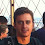## Sunday, June 19, 2011

### Tutorial: Electrical impedance made easy - Part 2

This is the second video of a short series in which I discuss the basics of electrical impedance from a practical standpoint. In this video, I explain the importance of knowing the magnitude and angle of impedance, as well as how this affects the power factor of a given electrical circuit.

1.One question on the 1K resistor. If it is designed to restrict the current when the circuit is switched on, is there any way to remove it from a running circuit to lower further the heat loss? It seems to me that having served its purpose it could be dialed to zero after power is applied.

2.LA Grant, yes that's a very insightful comment. In fact, resistors with a large negative temperature coefficient are used to limit in-rush current in many commercial designs. The resistor has high resistance when it is cold, but the resistance drops as the resistor heats up, thus making the circuit more efficient when running. There are other ways too, such as using active electronics to close a relay after the capacitors have charged through a resistor.

3.I think an inductor would also work to reduce the high frequency current when plugging it in, though according to my calculations it would take a 2.65H inductor, which wouldn't exactly be compact and would in fact have a significant real resistance anyway. If this were say, 100kHz it might be more practical.

It would also make a resonant circuit, a topic that might be worth its own video.

4.I think an inductor would also work to reduce the high frequency current when plugging it in, though according to my calculations it would take a 2.65H inductor, which wouldn't exactly be compact and would in fact have a significant real resistance anyway. If the line frequency were >50 kHz it would probably be more practical.

It would also make a resonant circuit, a topic that might be worth its own video.

5.Hi Ben,

In the video you show Power Factor = P/S where P is watts and S is the hypotenuse formed between P and I.

But when you did the power factor calculation, you calculated P/I (1100/5600) not P/S (1100/5707).. The results are pretty much the same (.19) but i wanted to confirm that it was cos(theta) not tan(90-theta)

Thanks for the tutorial!

Also it might be worth noting that power factor in practical terms is the ratio of the voltage to the current, eg a low power factor means current is drawn for a very small percentage of the time the voltage is high.

A power factor of .5 would be seen with a resistive load through a single diode, etc.

I really appreciate the way you bring the practical side out of the theory and i was surprised to see that part missing :)

-tmk

6.Robin, you are correct that inductors are rarely used for limiting in-rush current because they would need to be massive (thus expensive and bulky) to provide meaningful impedance. The DC resistance would also be somewhat high. Instead, designers sometimes use negative temperature coefficient resistors. These devices have high resistance when cold, but soon heat up, and drop their resistance to a low value. The hotter they get, the lower the resistance, so it is ideal for limiting in-rush current. For higher power designs, the circuit may use an active switch (relay, triac, etc) that shorts the current limiting resistor a short time after the device is powered-on.

7.Anonymous, I calculated P/S, which rounds to .19. P/Q would round to .2. Power factor is definitely the real power divided by the apparent power, which would be the cosine on the power graph.

Power factor indicates the phase difference between voltage and current. Thus, the power factor for a resistor/diode circuit would likely be 1. I am not positive about this, but I may test it myself since it is making me curious. Just because the circuit draws no power for half of the cycle, does not mean its power factor will drop. The only thing that will cause power factor to be less than 1 is reactance, which causes the current waveform to be out-of-phase with the voltage waveform. A resistor/diode circuit should have in-phase current and voltage for half the cycle, and no current for the other half, thus a power factor of 1.

8.Just re-watched it.. you were correct as usual, sorry for the trouble :)

-tmk

9.Just want to say thanks for this great video series!

10.Hi, just wanted to say thanks a lot... that was really well explained! I'd definitely like to hear more about impedance matching in e.g. audio cables!

11.This comment has been removed by the author.

12.Thank you for this clarifying tutorial! It helped me a lot! Im studying electronics by my self and this is really a subject that mustbe explained in a pratical manner, because it has very explicity pratical impacts. I´ve been trying to understand it theoricatically but its a lot harder that way... I hope you can post another video talking about impedance matching and more! Thanks!

13.Ben, very clear explanation. Will you please do a part 3?

14.These are great! The concepts are very well illustrated in a practical way, and I really like how you showed the difference between resistance and reactance with a physical example before jumping into the mathematical theory. I tutor physics and engineering courses at my school and this is a topic of confusion for many people; I would love to share these videos with students when we get to impedance this year. Looking forward to part 3!

15.The best tutorial on this subject I've ever seen. Part 3 please.

16.17.18.